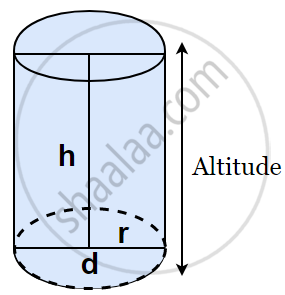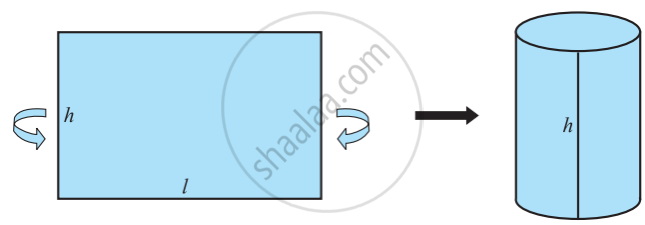# Surface Area of Cylinder

#### Topics

• Right Circular Cylinder
• Hollow Cylinder

## Definition

• Right Circular Cylinder: Right Circular Cylinder is a solid geometrical figure with straight parallel sides and a circular or oval cross-section.

## Formula

• Curved Surface Area of a Cylinder = 2πrh
• Total Surface Area of a Cylinder = 2πr(r + h)

# Right Circular Cylinder:

Right Circular Cylinder is a solid geometrical figure with straight parallel sides and a circular or oval cross-section.### Curved surface area of the cylinder:The area of the sheet gives us the curved surface area of the cylinder. Note that the length of the sheet is equal to the circumference of the circular base which is equal to 2πr.

So,

∴ Curved surface area of the cylinder = area of the rectangular sheet = length × breadth

= perimeter of the base of the cylinder × h

= 2πr × h

Therefore, Curved Surface Area of a Cylinder = 2πrh, where r is the radius of the base of the cylinder and h is the height of the cylinder.

# Total Surface Area of a Cylinder:If the top and the bottom of the cylinder are also to be covered, then we need two circles (in fact, circular regions) to do that, each of radius r, and thus having an area of πr 2 each, giving us the total surface area as 2πrh + 2πr 2 = 2πr(r + h).

So, Total Surface Area of a Cylinder = 2πr(r + h), where h is the height of the cylinder and r its radius.

## Example

In a building, there are 24 cylindrical pillars. For each pillar, the radius is 28 m, and the height is 4 m. Find the total cost of painting the curved surface area of the pillars at the rate of ₹ 8 per m2.

Radius of cylindrical pillar, r = 28 cm = 0.28 m, height = h = 4 m
curved surface area of a cylinder = 2πrh
curved surface area of a pillar = 2 xx 22/7 xx 0.28 xx 4 = 7.04 m2
curved surface area of 24 such pillar = 7.04 × 24 = 168.96 m2
cost of painting an area of 1 m2 = Rs. 8
Therefore, cost of painting 1689.6 m= 168.96 × 8 = Rs. 1351.68.

## Example

Find the height of a cylinder whose radius is 7 cm and the total surface area is 968 cm2.
Let height of the cylinder = h, radius = r = 7cm
Total surface area = 2πr(h + r)
i.e., 2 × 22/7 × 7 × (7 + h) = 968
h = 15 cm
Hence, the height of the cylinder is 15 cm.
If you would like to contribute notes or other learning material, please submit them using the button below.

### Shaalaa.com

Total Surface Area & Lateral Surface Area of a Cylinder [00:07:24]
S
0%